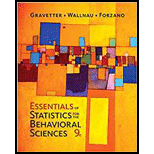# A researcher conducts an experiment comparing four treatment conditions with n - 12 scores in each condition. a. If the researcher uses an independent-measures design, how many individuals are needed for the study and what are the df values for the F-ratio? b. If the researcher uses a repeated-measures design, how many individuals are needed for the study and what are the df values for the F-ratio?### Essentials of Statistics for The B...

9th Edition
Frederick J Gravetter + 2 others
Publisher: Cengage Learning
ISBN: 9781337098120

#### Solutions

Chapter
Section### Essentials of Statistics for The B...

9th Edition
Frederick J Gravetter + 2 others
Publisher: Cengage Learning
ISBN: 9781337098120
Chapter 13, Problem 2P
Textbook Problem
9 views

## A researcher conducts an experiment comparing four treatment conditions with n - 12 scores in each condition. a. If the researcher uses an independent-measures design, how many individuals are needed for the study and what are the df values for the F-ratio? b. If the researcher uses a repeated-measures design, how many individuals are needed for the study and what are the df values for the F-ratio?

a.

To determine
The numbers of individuals and df values for the F-ratio needed for the study.

### Explanation of Solution

Given info:

A researcher conducts an experiment comparing four treatment conditions with n=12 scores in each condition in an independent-measure design.

Calculation:

Let n and k represent the numbers of participants in each treatment and total numbers of treatments. Then,

n=12k=4

In case of independent-measures design, participants in each treatment are different. Let, N represents total numbers of participants in the study, then,

N=n×k=12×4=<

b.

To determine
Numbers of individuals and df values for the F-ratio needed for the study.

### Still sussing out bartleby?

Check out a sample textbook solution.

See a sample solution

#### The Solution to Your Study Problems

Bartleby provides explanations to thousands of textbook problems written by our experts, many with advanced degrees!

Get Started

Find more solutions based on key concepts
Inequalities State whether each inequality is true or false. 35. (a) 3 4 (b) 3 4

Precalculus: Mathematics for Calculus (Standalone Book)

Find an equation of the tangent line to the curve at the given point. y=2x+1x+2,(1,1)

Single Variable Calculus: Early Transcendentals, Volume I

find the real roots of each equation by factoring. 133. 14x2x+1=0

Applied Calculus for the Managerial, Life, and Social Sciences: A Brief Approach

Evaluate without using a calculator. cos1(cos76)

Trigonometry (MindTap Course List)

For the area of the shaded region, which integral form, dx or dy, should be used? a) dx b) dy c) Either...

Study Guide for Stewart's Single Variable Calculus: Early Transcendentals, 8th

Rewritten in reverse order,

Study Guide for Stewart's Multivariable Calculus, 8th